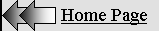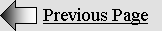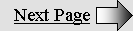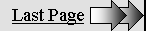LORENTZIAN  RELATIVITY

Page 1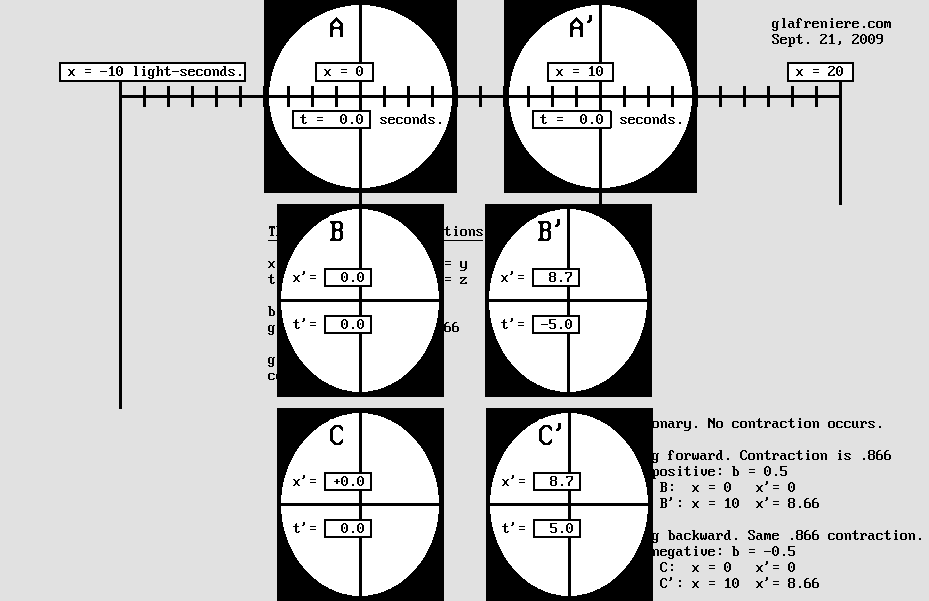Relativity is surprisingly simple.

It just requires elementary mathematical operations.

However, it also requires reasonable knowledge of the Lorentz Transformations.

This is why the above diagram should help.

THE LORENTZ TRANSFORMATIONS

The original Lorentz transformations were intended to cancel the Doppler effect on Maxwell's equations in a moving frame of reference. Lorentz and Poincare used them in order to recover the moving electron the way it appears in a stationary frame of reference. However, one may reverse Lorentz's equations (actually Poincare's simpler set using c = 1 and beta = v / c) to obtain the opposite effect:

x' =  g x + b t

t'  =  g t b x

y' = y      z' = z

b (beta) = v / c = 0.5

g (contraction) = Sqr(1 b ^ 2) = 0.866

This reversed equation set is much more easy to deal with because it yields exactly what Lorentz had in mind in 1904. See for yourself:

 x' =  g x + b t

1 – Matter undergoes a contraction in accordance with  g x. The normal A to A' distance being ten light-seconds, the B to B' distance below is 0.866 * 10 = 8.66 light-seconds instead. The 8 x 8 light-second squares transform into 8 x 6.9 rectangles, and circles transform to squashed ellipses. The contraction also applies to distances between pieces of matter for mechanical reasons.

2 – Matter moves from  g x  to  g x + b t. This is merely a translation motion, that is to say Galileo's transformation which leads to his well known Relativity Principle:  x' = x – v t, the reversed equation being:  x = x' + v t  or  x' = x + v t as well.

 t'  =  g t – b x

3 – Clocks display slower seconds in accordance with: t' = g t. Below, g = 0.866 so that clocks are displaying 8.66 slower seconds after a 10 second delay (.866 * 10 = 8.66).

4 – Clocks display a time shift in accordance with:  b x. Below, b (beta) = .5, so that the B' clock exhibits a –5 second time shift with respect to B according to –0.5 * 10.

Knowing that, one can easily compare the two systems A-A' and B-B' below: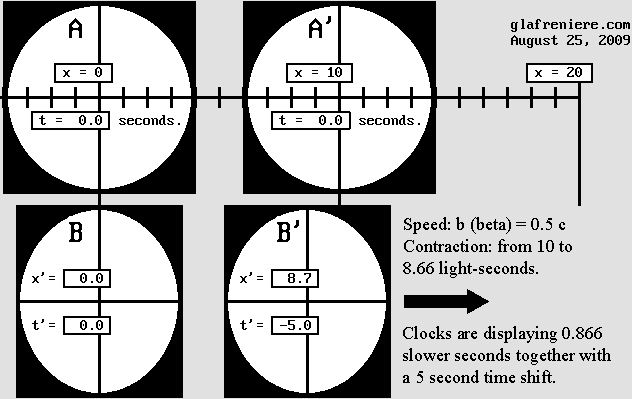Observers A and B adjust their clock to t = t' = 0 when they meet.

Observer B' then tries to synchronize his clock according to B, but the procedure ends up with a 5 second time shift.

Observers B and B' try to maintain a 10 light-second distance to each other but the resulting distance is rather 8.66 light-seconds.

Now, let's examine the situation after a 20 second delay: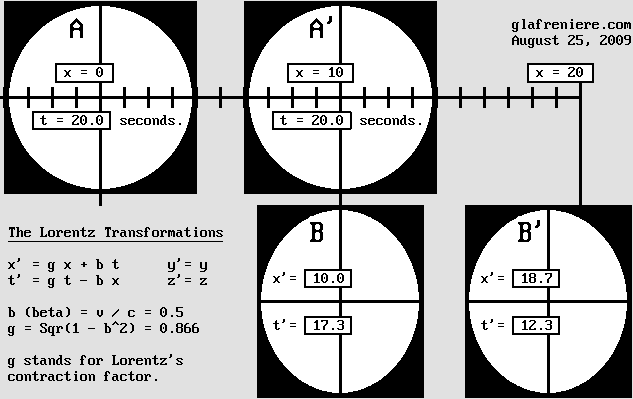Because observer B truly moves at 0.5 c, he is now meeting exactly A' whose distance to A is 10 light-seconds.

Because clock B ticks slower, it displays only: g * t = 17.32 seconds instead of 20 seconds.

Observer B is unaware of the contraction. Additionally, he erroneously observes that A and A' are moving backward at 0.5 c.

B erroneously observes that clock A is ticking slower so that it now seems to display: g * 17.32 = 15 seconds.

Because he is meeting A', B observes that the apparently slower clock A' exhibits a + 5 second time shift (15 + 5 = 20 sec).

B thinks that his own clock indicates 17.3 seconds because apparently, the A to A' distance is only 8.66 light-seconds.

Observer B comes to the conclusion that he is stationary and that A and A' are transformed and moving leftward at 0.5 c.

Because his measures are correct, observer A also comes to the conclusion that he is stationary and that B and B' are moving.

 In the end, nobody can say for sure which one is moving. This phenomenon is all about Lorentz's Relativity. Why make things so complicated?

Below is a video which reproduces more clearly the scene shown above. It should be helpful in order to understand why observer B especially is fooled by the Lorentz transformations.

Lorentz_Transformations.mkv

Lorentz's reversed equations.

You may doubt my equations, which is advisable. One must firstly be aware that Henri Poincare discovered Relativity well before Einstein and that he was a superb mathematician. He could simplify Lorentz's equations (Woldemar Voigt's ones, actually) as soon as 1901. Here is a copy from his French book "Electricity and Optics":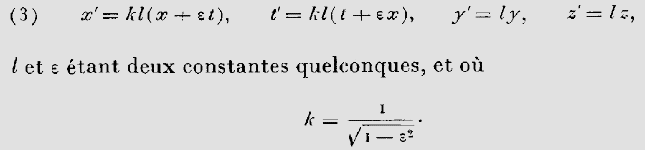Poincare could simplify Woldemar Voigt's equations as shown above, but Voigt's useless constant "l" is still present.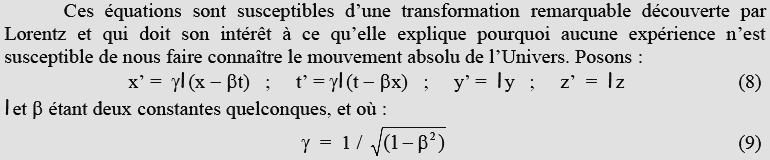This is a transposition by Christian Marchal using more modern notation such as the gamma factor and the beta normalized speed.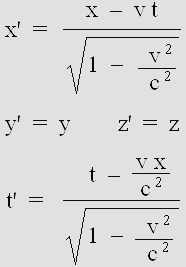By comparison, Lorentz original equations appear quite obscure.

The first equation may be simplified to: x' = (x v t) / g  and Voigt's useless constant is absent.

Unfortunately, this equation set creates a new "space-time" frame of reference which is incompatible with the first one.

Lorentz found that Voigt's constant was useless because its value should be 1. This constant being eliminated, Lorentz's first equation simplifies to:

According to Lorentz :  x' = (x v t) / g

According to Poincare :  x' =  gamma (x b t)

The unknown variable being x, Poincare's equation must be rearranged like this:

x = x' / gamma + b t

Then Lorentz's contraction factor g, which is given by 1 / gamma,  may replace the gamma factor:

x = g x' + b t

And finally, because x and t should obviously stand for the unmoving system variables, one must swap x and x':

 x' =  g x + b t

The same process applied to the time equation leads to:

 t'  =  g t – b x

Thus, and this is true even for Einstein's Relativity,  x' for the moving observer B' shown above is 18.66 light-seconds, and t' is 12.32 seconds after a 20 second delay (x = 10, t = 20, beta = 0.5,  g = 0.866):

x' = g x + b t = (0.866 * 10) + (0.5 * 20) =  18.66 light-seconds.

t' = g t b x = (0.866 * 20) (0.5 * 10) = 12.32 seconds.

What is remarkable is that all those variables (x, x'; t, t') along with the sign ( instead of +) are fully reversible in order to retrieve the initial x and t variables. This amazing reciprocity was Poincare's discovery, who used it in 1904 in order to establish his Relativity Postulate:

x = g x' b t' = 10 light-seconds.

t = g t' + b x' = 20 seconds.

Lorentz's version also works on the condition that x and x' are swapped (hence using t, not t'):

x = (x' b t) / g = 10 secondes-lumière.

It should be pointed out that these new inverted equations are mainly intended to transform a stationary emitter into a moving one. This produces a Doppler effect. In this case, the Cartesian coordinates x, y et z stand for wavelength and the t variable stands for the wave period in 2 * pi units. Their purpose is definitely not to transform space and time. The original version was supposed to correct the Doppler effect instead, and this is exactly what Woldemar Voigt had in mind in 1887.

However, it becomes clear that Voigt's initial equations were wrong for many reasons. His constant was discarded by Lorentz, but it may still be useful in order to obtain the normal Doppler effect. In this case, its value equals g and it should divide the product g * t in the t' equation, not multiply it (compare Poincare's version above to my version below). It was a serious error. In addition, one must use the plus sign for the x equation and the minus sign for the t' one, yet this may be just an anomaly related to Maxwell's equations. And finally, because the x and t variables stand for the moving system coordinates, the original equation set creates an unnecessary and incompatible new frame of reference. Below is the corrected and inverted equation set: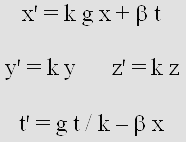This is Woldemar Voigt's revisited equation set.

It remains useful because it can produce a variable Doppler effect where the frequency is adjustable.

In the case of the normal Doppler effect, the k constant equals g and the frequency is unchanged.

In the case of light and radio waves, the k constant value is 1 and the frequency is slowed down according to g.

Consequently, any Doppler effect involving light or radio waves such as those emitted by a satellite supposes that the frequency is slower according to g, unless it is corrected afterwards. It is undoubtedly a relativistic effect. For example, if  the observer is traveling inside the satellite, he is totally unable to detect the Doppler effect in spite of the fact that it is easily detectable on the earth's surface. This occurs because he himself is transformed according to Lorentz.

It is about the Doppler effect.

Those facts are undisputable. Lorentz's formulas just produce or correct a Doppler effect. And because they were wrongly used as a mathematical artifice whose effect was to transform space and time, I had to correct them. The equation set shown below is simply about a special Doppler effect involving a slower frequency. It just transforms the wavelength and the wave period, not space and time. It is not a theory, is is a fact which is easily verifiable on any emitter surrounded by spherical waves.

I wrote a program showing this:

Doppler_Voigt_transformations.bas      Doppler _Voigt_transformations.exe

I did not remove Voigt's constant and hence, you have a choice of four different frequencies. You may press A, B, C or D to change the value of the constant. In Lorentz's version the k =1 constant does not appear any more. In this case, one obtains y' = y and z' = z, and the emitter frequency slows down according to g so that there is no transverse wavelength contraction. Please examine the source code to verify that I am really using my own version of Voigt's equations.

This is why I propose that Lorentz's equations should be corrected as below. The reversed set on the right was obtained using Poincare's method: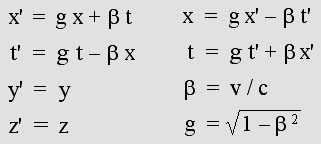The Lorentz Transformations revisited.

The x et x' variables apply to the same Cartesian frame of reference, which remains stationary.

Please bear in mind that this reversed equation set yields totally new results.

Strangely, those new results are still consistent with those predicted by Lorentz, Poincare and Einstein.

As a matter of fact, they all predicted that a moving material body should appear shorter according to g * x.

It should also move according to beta * t. Thus, the equation x' = g x + b t is no surprise.

And finally, Lorentz, Poincare and Einstein also told us that a moving clock should tick slower according to: g * t.

The accurate time shift according to beta * x  or v * x / c^2 was Lorentz's discovery.

In spite of that, nobody ever proposed those equations up to now. This is weird.

The Time Scanner performs even better.

Please examine this small video which I very proud of. It is honestly amazing. It contains a lot of information showing that Lorentz's version of Relativity (1904) was correct.

Time_Scanner.mkv

It is a high definition (1280 x 720) Mpeg-4 video using the now well accepted H-264 standard (Divx-7 .mkv Matroska file). I suggest VLC Media Player and the whole bunch of CCCP codecs (unrelated to the USSR!) which includes a free version of Zoom Player, also an excellent player. Below is the FreeBasic program which produced the images.

WaveMechanics07.bas

My Time Scanner is capable of accelerating the whole system where emitters and material bodies are moving at different speeds, on condition that they are already transformed. Here, it is accelerated to 50% of the speed of light to the right. However, C and C' are already moving to the right at this speed, and hence their new speed should be the speed of light. But Lorentz and Poincare discovered that no material body is capable of reaching the speed of light. This is why their resulting speed is only .8 c, according to Poincare's law of speed addition which is given by:

beta'' = (beta + beta') / (1 + beta * beta')

In my opinion, such a magnificent harmony should leave nobody unfeeling. Now, thanks to Lorentz, Relativity can be explained. There are no paradoxes any more. All is clear and logical.

On the one hand, it becomes possible to display more than two material bodies (hundreds, actually) whose speed and direction may differ. The results remain perfectly coherent after the scanning process, or the acceleration if you prefer.

On the other hand, a preferred stationary frame of reference may be designated as a convention. It should allow all observers to have a better image of their situation. Ideally, it is the one whose speed is intermediate, for instance the red scale as seen from A and B. Surprisingly, this red scale does not transform because its intermediate speed (not exactly .25 c because of Poincare's law of speed addition) is simply inverted after undergoing the acceleration. Its speed being the same, its contraction remains the same, so that A and B can adjust its length when they meet (they must consider that they are moving in opposite directions at the same speed). They can also adjust its clock rate so that it stubbornly displays the "absolute" time on condition that is is placed where x = 0 when A and B meet and on condition that they synchronize all clocks according to t = 0 when they meet, too. The time shift is inverted everywhere else because the red scale is stopped, then accelerated at the same speed in the opposite direction.

All becomes perfectly clear. The red scale length does not change and the clock displays the absolute time on condition that A and B,  A and C, or B and C (two of them only) designate an intermediate one as a preferred Cartesian frame of reference. Thus, there exists a situation in which space and time remain constant. To put it even more clearly, as Euclid, Galileo, Descartes and Newton always thought, space and time do not transform.

In practice, Einstein's theory of Relativity appears inapplicable. Establishing a preferred frame of reference is advantageous. The present demonstration indicates that many of them are possible but that some of them are much better. Only Lorentz's version of Relativity allows such a choice. It should be pointed out that all this cannot be explained without the presence of the aether. Even if it cannot be detected, all happens as if it exists. It is imperative that the speed of light is related to it.

The Lorentz transformations indicate that all this happens solely because of the Doppler effect. This strongly suggests that matter is undergoing the Doppler effect. Matter behaves like waves do. Lorentz's Relativity indicates that matter consists of waves propagating at the speed of light. Such waves need a medium, the aether, so that finally, our material world is nothing but the aether...

It turns out that the Lorentz transformations are leading us well beyond Relativity. Now, we are dealing with matter mechanics, more accurately the Wave Mechanics.

The conclusion is that Newton's laws as well as all physical phenomena descriptions must be upgraded in order to conform with the Wave Mechanics.

LORENTZIAN RELATIVITY IS THE ONLY CORRECT ONE

The goal of this page is to show that Lorentz's Relativity is easily explainable. It becomes possible to show why such phenomena are possible. Unfortunately, Lorentz never released a complete version of his ideas like Einstein did. More or less recently, many authors tried to promote them but they frequently deviate into erratic opinions such as faster-than-light speeds. They obviously forget that Lorentz himself found that inertia, and hence matter mass increases according to its speed in such a way that it would be infinite at the speed of light.

This is why I am explaining Lorentz version of Relativity the way he himself would have done. As far as I know, this page is fully compatible with Lorentz's ideas, at least around 1904. It is by no means revolutionary.

It should be emphasized that Lorentz never doubted the existence of an aether until his death, which occurred in 1928, and hence many years after Einstein's theory triumphed. Unlike Poincare, he was sure that there should exist a preferred frame of reference. He was convinced that matter truly contracts and that moving clocks are displaying slower seconds along with a "local time". Such clocks cannot show the "true time".

To put it clearer, Lorentz never proposed an absurd "space-time" transformation. Poincare was much less rigorous. He rejected matter contraction. He thought that optical phenomena were only relative and he wrote that Lorentz's hypothesis was not satisfactory. He even wrote that the aether might some day be considered useless.

Below are two quotes from Lorentz which were written several years after 1904. They indicate that Lorentz had finally given up his previous concept and accepted much of Poincare's and Einstein's ideas. However, they are very clear on Lorentz's 1904 theory:

 I had not thought of the straight path leading to them, since I considered there was an essential difference between the reference systems x, y, z, t and x', y', z', t'. In one of them were used - such was my reasoning - coordinate axes with a definite position in ether and what could be termed true time; in the other, on the contrary, one simply dealt with auxiliary quantities introduced with the aid of a mathematical trick. The chief cause of my failure was my clinging to the idea that the variable t only can be considered as the true time and that my local time t' must be regarded as no more than an auxiliary mathematical quantity.

According to Lorentz, x' and t' are only auxiliary quantities introduced with the aid of a mathematical trick.

The variable t only can be considered as the "true time".

THE SCIENCE OF ILLUSION

Relativity is all about mystification where the Doppler effect hides from our eyes what is really going on. This can easily be explained on the condition that matter and fields of force are made of standing waves, and that all forces such as light and gravity are also caused by waves.

Clearly, any moving wave system should undergo the Doppler effect. However it is not that simple because the regular Doppler effect is asymmetric. For example, a bell moving at beta = sin 30° = .5 times the speed of sound emits sound waves which are lengthened backward less than they are compressed forward:

The forward wavelength is:  1 beta  =  .5 time shorter.

The backward wavelength is:  1 + beta  =  1.5 times longer.

Additionally, in the presence of a strong wind or in a moving frame of reference such as a train platform, additional effects occur because the observer is moving with respect to air. He may use a moving source and a moving screen in order to produce standing waves and check for the first node position (this is called the Hertz test).

Then the transverse wavelength and the transverse standing wave pattern are:

g  =  cos 30°  =  sqr(1 beta ^ 2)  =  .866 time shorter.

However, the moving axial standing wave pattern is:

g ^ 2  =  .75 time shorter.

Thus the difference allows the moving observer to detect his speed as compared to the wave medium. But this is no longer possible for matter or forces such as gravity and light because Lorentz's Doppler effect is perfectly symmetrical.

The point is that any periodic phenomenon slows down at high speed in accordance with the contraction factor: g = sqr(1 beta^2). This was well established by Hendrick A. Lorentz in 1904. More particularly, the electron frequency slows down according to g:

 F' = g F

The electron frequency slows down according to Lorentz's contraction factor.

This phenomenon alone causes the Lorentz transformations and explains Relativity.

Surprisingly, the frequency reduction produces a perfectly symmetric Doppler effect as seen by an unmoving observer. As compared to waves in an unmoving system, the contraction forward and the dilation backward occur according to the same ratio.

Let us suppose that the velocity of a complex wave system such as the electron is half of the speed of light, so that beta = .5 and g = .866. The forward or backward frequency ratio is R = (1 + beta) / g  =  1.732.

The forward wavelength is:  (1 beta) / g  =  1 / R  =  .577  time shorter.

The backward wavelength is:  (1 + beta) / g  =  R  =  1.732  times longer.

The transverse wavelength is:  g / g  =  1  (finally unchanged).

The transverse standing wave pattern is:  g / g  =  1  (finally unchanged).

The moving axial standing wave pattern is:  g ^ 2 / g  =  g  =  .866  time shorter.

The axial standing wave node and antinode pattern becomes g = .866 shorter instead of g squared = .75. The point is that the electron is made of standing waves. It is a well known fact that the 8-electron atomic external layer radius, which is responsible for chemical binding, is linked to a given wavelength. This explains why molecules, hence matter, undergo an axial contraction according to g only instead of g squared.

Please note the incredible symmetry:  1 / 1.732 = .577 and inversely,  1 / .577 = 1.732 according to the Doppler ratio  R = (1 + beta) / g  =  1.732  >  1.

Detecting relative motion only.

On the one hand, this symmetry requires the observer to use the same R ratio for the redshift and the blueshift from matter or galaxies moving away from him or towards him (albeit the blueshift rarely occurs). On the other hand, the results would be exactly the same if the galaxy was rather stationary and if the observer was moving away from it or towards it. Whatever the situation, he does not have a choice any more. In both cases, he must use the formula below (lambda stands for wavelength as measured in his frame of reference) in order to establish the galaxy's beta velocity.

Thus, he will obtain only the relative velocity, which is given by: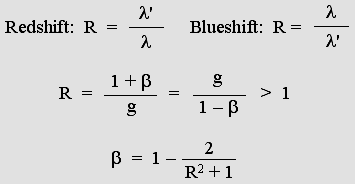Considering those results, the observer should be aware that this galaxy could stand perfectly still as well. He himself would rather be moving away at .866 c and, in this case, his whole environment would be transformed according to Lorentz. Especially, clocks would run two times slower; in this example they would display just .5 second instead of one absolute second.

The light waves emitted by the unmoving galaxy are free from any frequency reduction and any Doppler effect, but the moving observer experiences the virtual Doppler effect according to f ' = f (1 beta) = .13397 time the original frequency. Additionally, his clocks indicate slower hours and the recorded frequency will seem to be .13397 / .5 = .26794 as compared to the original and absolute one, hence a perfectly identical and symmetric redshift ratio: R = 1 / .26794 = 3.732.

So, simply because the electron frequency slows down at high speed, the blueshift vs. redshift ratio can no longer inform the observer whether he is moving or not. Actually, both the observer and the galaxy are most likely to be moving with respect to the aether, but their absolute velocity can never be established. Whatever the situation, recorded data indicates relative velocity only.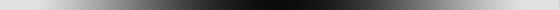Hundreds of examples.

One may carefully examine a lot of experiments where Lorentz's Relativity holds true. The list below is not exhaustive. Fortunately, they do not require complicated formulas and so many successful demonstrations as a whole finally builds a solid proof that Lorentz was right.

1. The absence of transverse contraction prevents the observer from detecting his absolute motion.

2. The radar echoes simulate or dissimulate the axial contraction.

3. The clock synchronization procedure ends up with a time shift.

4. The absolute motion causes the slowing down of all mechanisms.

5. The contraction cannot be detected by any means such as optical imaging or triangulation.

6. The speed of light seems to be the same in all frames of reference.

7. There are no means to detect that clocks are running slower.

8. The Michelson interferometer cannot detect its absolute motion because it undergoes a contraction.

9. The beam splitter angle must match the interferometer contraction in order to reflect correctly the light beam.

10. Bradley's stellar aberration cannot reveal the Earth's absolute motion.

11. The Fresnel-Fraunhofer diffraction pattern cannot reveal the absolute motion.

12. Light undergoes a symmetrical Doppler effect, so that relative motion only can be detected.

13. Any speed beyond the speed of light is impossible.

14. The relative speed between two material bodies may approach twice the speed of lignt.

15. Standing waves contract according to Lorentz on the condition that the frequency also slows down according to Lorentz.

16. Lorentzian Relativity remains consistent in the presence of an unlimited number of material bodies.

17. There is no General Relativity because gravity is not linked to Relativity.

18. The Lorentz transformations lead to Relativity but they also extend well beyond it.

This will be examined in the next page.| 01 | 02 | 03 | 04 | 05 | 06 | 07 | 08 | 09 | 10 | You are here. | 12 | 13 | 14 | 15 | 16 |

| 17 | 18 | 19 | 20 | 21 | 22 | 23 | 24 | 25 | 26 | 27 | 28 | 29 | 30 | 31 | 32 | 33 |

 Gabriel LaFreniere, Bois-des-Filion in Québec. Email: Please read this notice. On the Internet since September 2002. Last update December 3, 2009.